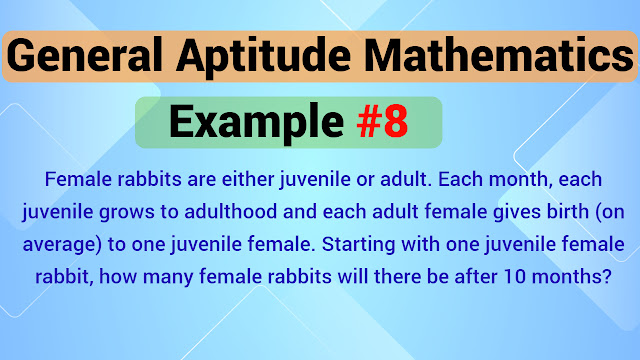# Female rabbits are either juvenile or adult. Each month, each juvenile grows to adulthood and each adult female gives birth (on average) to one juvenile female. Starting with one juvenile female rabbit, how many female rabbits will there be after 10 months?

##Question:

Female rabbits are either juvenile or adult. Each month, each juvenile grows to adulthood and each adult female gives birth (on average) to one juvenile female. Starting with one juvenile female rabbit, how many female rabbits will there be after 10 months?
1) 71
2) 79
3) 81
4) 89

We start with one juvenile female at the begining of the $1^{st}$ month.
At the end of the $1^{st}$ month that juvenile will turn into an adult female.
So we have 1 (1 adult) female rabit at the end of $1^{st}$ month.
As per conditions given in the example, this 1 adult female rabbit will give birth to 1 juvenile female rabbit.
So at the end of $2^{nd}$ month there will be 2 (1 juvenile, 1 adult) female rabbits.
Now, in $3^{rd}$ month, 2 female rabbits at the end of the $2^{nd}$ month will be there and in addition to that one of it adult female will give birth to the juvenile female.
Here, the number of female rabbits at the end of each month follows Fibbonachi sequence.
i.e. the number of female rabbits at the end of $n^{th}$ month will be the sum of number of female rabbits at the end of $(n-1)^{th}$ month number of female rabbits at the end of $(n-2)^{th}$ month.
$\therefore$ following are the details of number of female rabbits at the end of each month:
$0^{th}$ month = 1
$1^{st}$ month = 1
$2^{nd}$ month = 2
$3^{rd}$ month = 3
$4^{th}$ month = 5
$5^{th}$ month = 8
$6^{th}$ month = 13
$7^{th}$ month = 21
$8^{th}$ month = 34
$9^{th}$ month = 55
$10^{th}$ month = 89